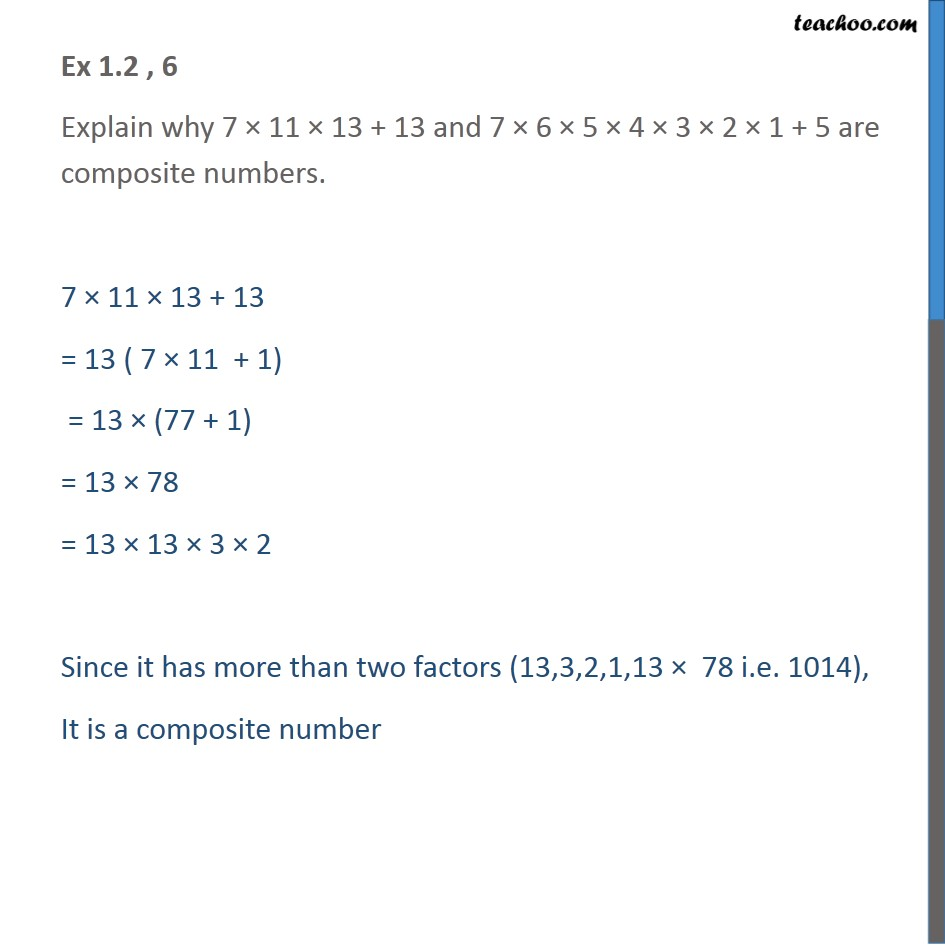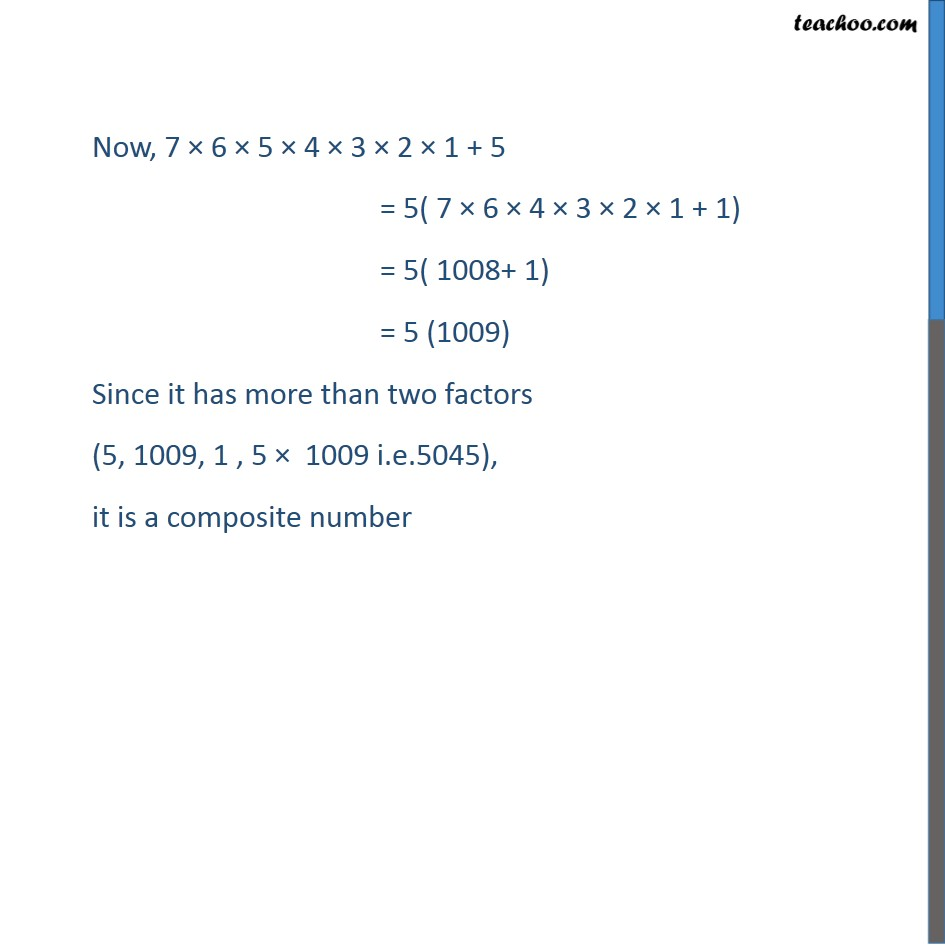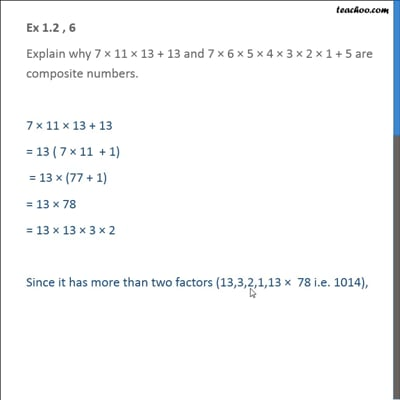Ex 1.2

Chapter 1 Class 10 Real Numbers (Term 1)
Serial order wiseThis video is only available for Teachoo black users

### Transcript

Ex 1.2 , 6 Explain why 7 × 11 × 13 + 13 and 7 × 6 × 5 × 4 × 3 × 2 × 1 + 5 are composite numbers. 7 × 11 × 13 + 13 = 13 ( 7 × 11 + 1) = 13 × (77 + 1) = 13 × 78 = 13 × 13 × 3 × 2 Since it has more than two factors (13,3,2,1,13 × 78 i.e. 1014), It is a composite number Now, 7 × 6 × 5 × 4 × 3 × 2 × 1 + 5 = 5( 7 × 6 × 4 × 3 × 2 × 1 + 1) = 5( 1008+ 1) = 5 (1009) Since it has more than two factors (5, 1009, 1 , 5 × 1009 i.e.5045), it is a composite number